# Merge table records based on unique field, but overwrite with specific attributes

500
2
Jump to solution
05-01-2017 08:46 AMNew Contributor III

I have a table of records that includes categorical probabilities (Low, Medium, High) for species within 'zones' as represented by a unique id. See below for example: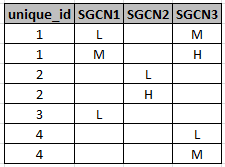The table has a one to many relationship for zones to probability if there is more than one probability of a species within a zone (i.e. a zone could be marked with L and M, in which case there would be two records for the zone).

I would like to flatten the zones based on the unique id and overwrite the probability with the largest probability (so, H would always overwrite M or L, and M would always overwrite L, and L would always overwrite blanks), so the table above would end up looking like this: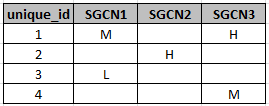I am using ArcMap 10.4 and am also using Arcpy for some processing.  I don't think this can be done using merge because there is not an option to overwrite based on specific attributes. I imagine this may be able to be done with arcpy using cursors, but I have no idea where to start with cursors for this specific outcome. Can someone point me in the right direction with this or let me know if there is a better method?

1 Solution

Accepted Solutions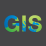byEsri Esteemed Contributor

Hi Molly,

Here is one way you could do this.

1.  Use the Field Calculator to convert the L, M, H values to integer.  That will allow you to run the 'Summary Statistics' tool and return only the 'MAX' value for that unique id.  Here is an example that you would run on each field changing !SGCN1! to !SGCN2! and to !SGCN2! for the second dialog.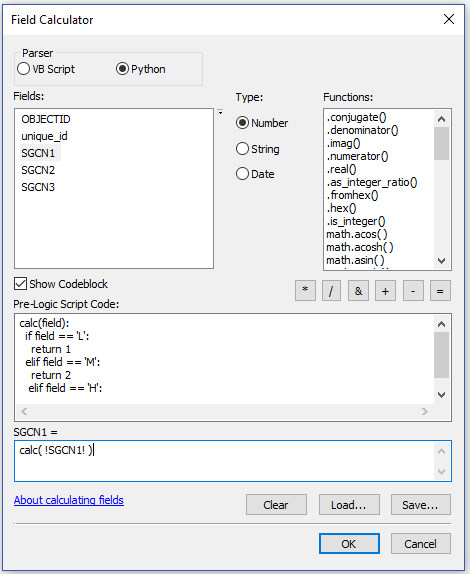2.  Run the Summary Statistics tool specifying the 'MAX' for each field and unique_id for the Case Field: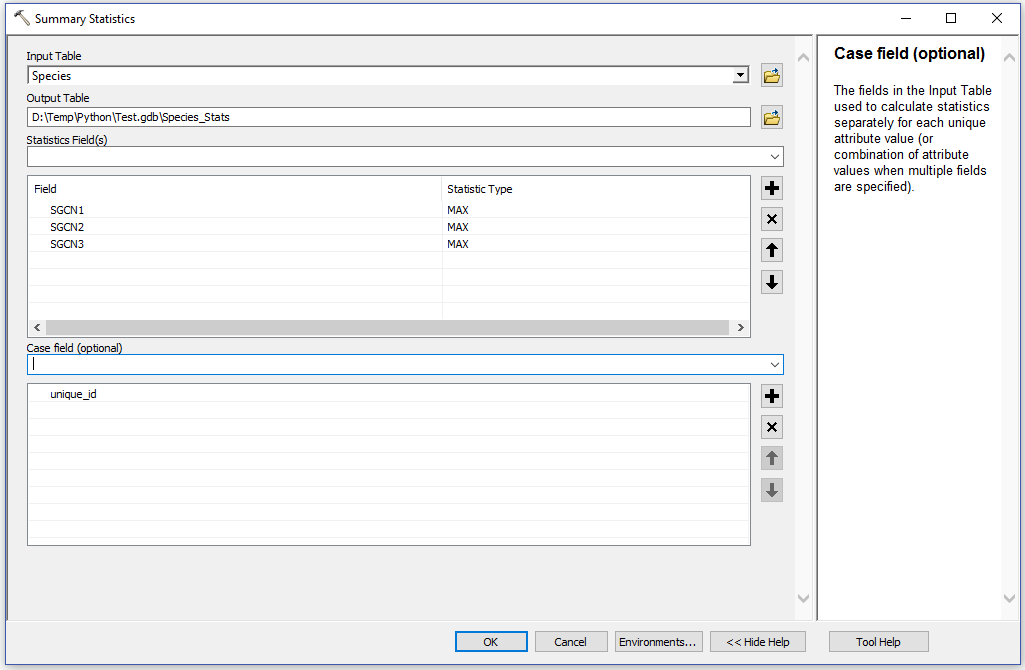3.  You can then convert the integers back to text using the Field Calculator: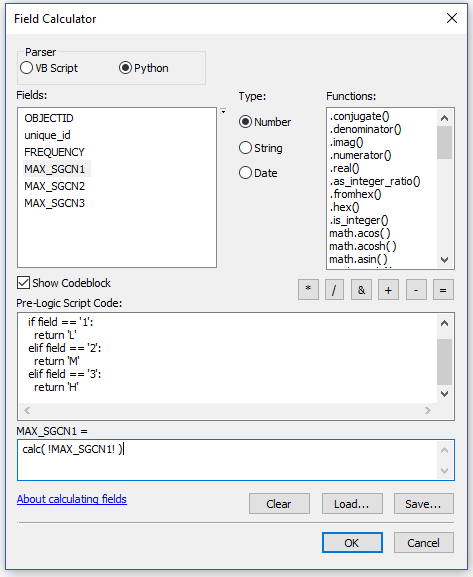2 RepliesbyEsri Esteemed Contributor

Hi Molly,

Here is one way you could do this.

1.  Use the Field Calculator to convert the L, M, H values to integer.  That will allow you to run the 'Summary Statistics' tool and return only the 'MAX' value for that unique id.  Here is an example that you would run on each field changing !SGCN1! to !SGCN2! and to !SGCN2! for the second dialog.2.  Run the Summary Statistics tool specifying the 'MAX' for each field and unique_id for the Case Field:3.  You can then convert the integers back to text using the Field Calculator:New Contributor III

Hi Jake,

Great, this will work.  I went through manually to check and am going to write it into a script next.  Thanks for the suggestions!